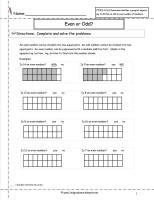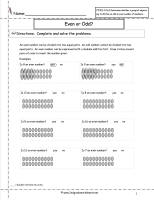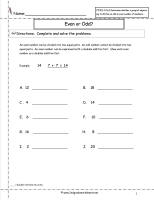﻿ CCSS 2.OA.3 Worksheets

# Common Core State Standard 2.OA.3

## Operations & Algebraic Thinking

### Work with equal groups of objects to gain foundations for multiplication.

3. Determine whether a group of objects (up to 20) has an odd or even number of members, e.g., by pairing objects or counting them by 2s; write an equation to express an even number as a sum of two equal addends.

###Odd or Even Worksheet

Common Core State Standards: CCSS 2.OA.3
Students must determine if given numbers are odd or even by shading boxes.  Students determine even numbers by seeing if the number can be placed into two equal groups.

###Odd or Even Worksheets

Common Core State Standards: CCSS 2.OA.3
Students must determine if given numbers are odd or even by circling pairs of ovals.  Students determine even numbers by seeing if the number can be placed into two equal groups.

###Odd or Even Worksheet

Common Core State Standards: CCSS 2.OA.3
Even numbers can be represented by a doubles addition fact.  Students represent each even number in a doubles addition fact.

###Odd or Even Worksheets

Common Core State Standards: CCSS 2.OA.3 Primer, Prerequisite

Various worksheets dealing with odd and even numbers.